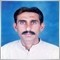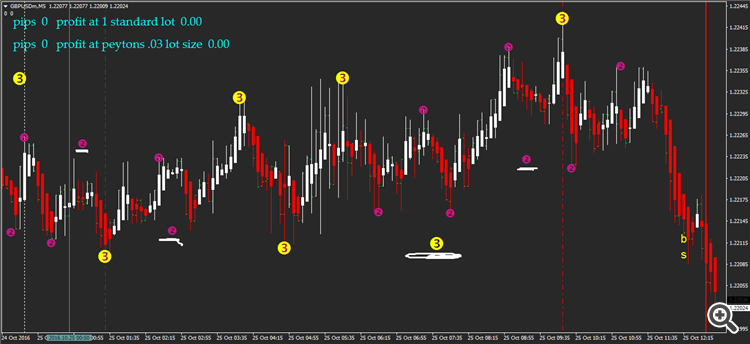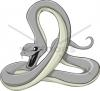Elite indicators :) - page 1381527

mntiwana:

Thanks for confirm this but i was just means because of its smoothing factor that can form ha an late signaling indicator.is not that ?

regards

If you're using HAMA, you'll get late signal - more late than usual HA.

But in time like today, GU will probably survive with HA trading + semafor. You can put any indicator you want, but with HA it is sufficient (at least for me).

HA, unlike any other indicators doesn't have parameter to be set on. You only can choose color if that's the problem.

I'll show you some tricky trades that were made using HA+semafor.4527

redwed:
If you're using HAMA, you'll get late signal - more late than usual HA.

But in time like today, GU will probably survive with HA trading + semafor. You can put any indicator you want, but with HA it is sufficient (at least for me).

HA, unlike any other indicators doesn't have parameter to be set on. You only can choose color if that's the problem.

I'll show you some tricky trades that were made using HA+semafor.
Of course,ha is simple to use,and your tricks help users to format their decisions :)527

Smoothing adds lag, that is true. It only depends what are we looking for (lag is not always bad - after all, in some cases, only lag is what filters out the false signals) but that is the eternal story of what we are looking for :)
Hello sir. Can you find any faults in this indicator? They paint in wrong order.Files:151106

redwed:
Hello sir. Can you find any faults in this indicator? They paint in wrong order.

ATTENTION: Video should be reuploaded

They are working OK. They are simply drawing those signs at a place where they are drawn at the higher time frame (since you are probably using it in multi time frame mode)527

They are working OK. They are simply drawing those signs at a place where they are drawn at the higher time frame (since you are probably using it in multi time frame mode)
No im not. I don't adjust the tf just yet. In fact, i put in the fresh setting for this chart.151106

redwed:
No im not. I don't adjust the tf just yet. In fact, i put in the fresh setting for this chart.

No idea why it draws it like that. The code how it calculates is as the original author made it - so only he can know why jhe made it that way143

I have a question and a request. Would it be possible to code the FRAMA indicator. I found one on the tradingview website.

Here is the code:

//@version=2

study("Fractal Adaptive Moving Average",shorttitle="FRAMA",overlay=true)

price = close

len = input(defval=252,minval=1)

FC = input(defval=40,minval=1)

SC = input(defval=252,minval=1)

w = log(2/(SC+1))

len1 = len/2

H1 = highest(high,len1)

L1 = lowest(low,len1)

N1 = (H1 - L1)/len1

H2 = for i = len1+1 to len

high>high[i]?high:high[i]

L2 = for i = len1+1 to len

low>low[i]?low:low[i]

N2 = (H2 - L2)/len1

H3 = highest(high,len)

L3 = lowest(low,len)

N3 = (H1 - L1)/len

dimen = (log(H1+H2) - log(H3))/log(2)

oldalpha = exp(w*(dimen-1))

oldN = (2-oldalpha)/oldalpha

newN = ((SC-FC)*(oldN-1)/(SC-1))+FC

alpha = 2/(newN+1)

out = nz(out)*(1-alpha) + price*alpha

plot(out,title="FRAMA")

This will probably not much of use, but can you code this?

Thx

D.151106

Desatar:

I have a question and a request. Would it be possible to code the FRAMA indicator. I found one on the tradingview website.

Here is the code:

//@version=2

study("Fractal Adaptive Moving Average",shorttitle="FRAMA",overlay=true)

price = close

len = input(defval=252,minval=1)

FC = input(defval=40,minval=1)

SC = input(defval=252,minval=1)

w = log(2/(SC+1))

len1 = len/2

H1 = highest(high,len1)

L1 = lowest(low,len1)

N1 = (H1 - L1)/len1

H2 = for i = len1+1 to len

high>high[i]?high:high[i]

L2 = for i = len1+1 to len

low>low[i]?low:low[i]

N2 = (H2 - L2)/len1

H3 = highest(high,len)

L3 = lowest(low,len)

N3 = (H1 - L1)/len

dimen = (log(H1+H2) - log(H3))/log(2)

oldalpha = exp(w*(dimen-1))

oldN = (2-oldalpha)/oldalpha

newN = ((SC-FC)*(oldN-1)/(SC-1))+FC

alpha = 2/(newN+1)

out = nz(out)*(1-alpha) + price*alpha

plot(out,title="FRAMA")

This will probably not much of use, but can you code this?

Thx

D.

You already have a frama indicator (for a long time) for metatrader

Here is just one version (a bit more usable than the single value version - multi time frame, filters alerts and all the bells and whistles included) : https://www.mql5.com/en/forum/178397/page152143

Hello,

thanx for the fast reply.

I saw on a testing website the following settings for the frama that I can not replicate with your indicator. Or maybe different inputs are nessecary.

The website is http://etfhq.com/blog/2010/10/09/frama-is-it-effective/#Best.

Can you have a look?

Thx.

D.

Files:
Frama.JPG 56 kb186